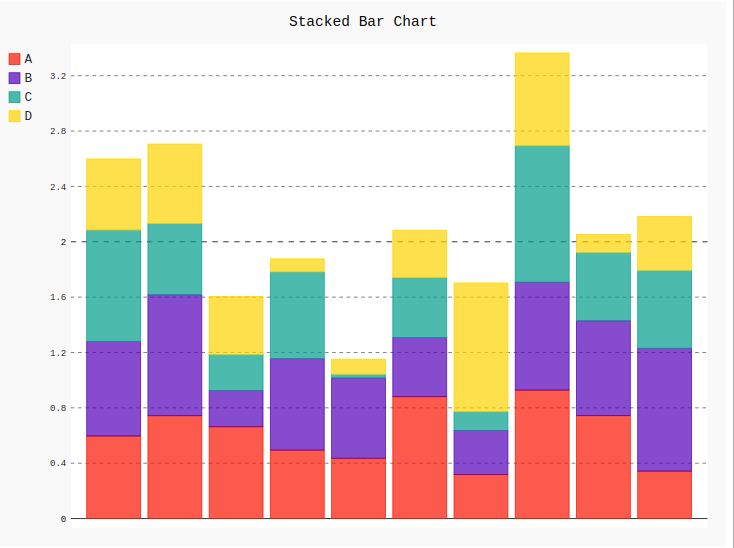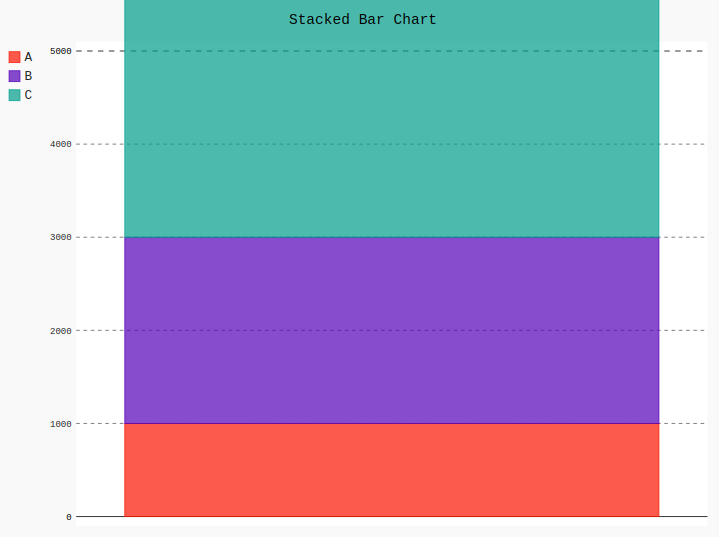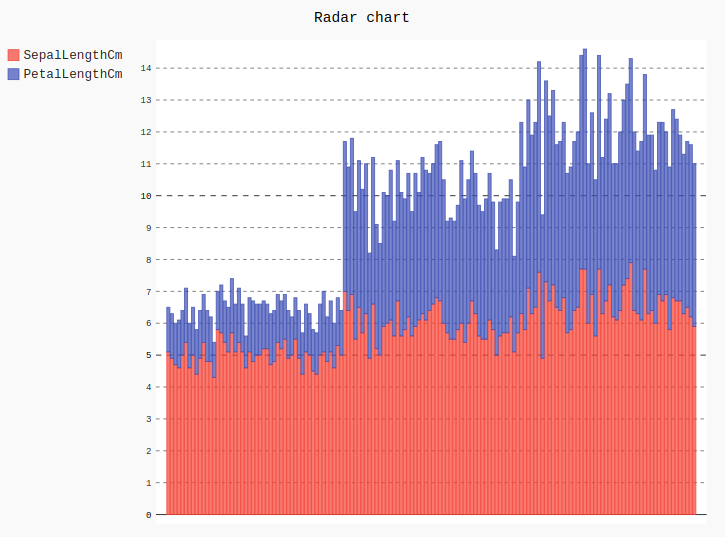# Stacked Bar chart in pygal

Pygal is a Python module that is mainly used to build SVG (Scalar Vector Graphics) graphs and charts. SVG is a vector-based graphics in the XML format that can be edited in any editor. Pygal can create graphs with minimal lines of code that can be easy to understand and write.

## Stacked Bar Chart

This graph is similar to the bar chart but the only difference is that the values are stacked in it. It can be created using the StackedBar() method.

Syntax:

```line_chart = pygal.StackedBar()
```

Example 1:

## Python3

 `# importing pygal ` `import` `pygal ` `import` `numpy ` ` `  ` `  `# creating the chart object ` `bar_chart ``=` `pygal.StackedBar() ` ` `  `# naming the title ` `bar_chart.title ``=` `'Stacked Bar Chart'`         ` `  `# Random data ` `bar_chart.add(``'A'``, numpy.random.rand(``10``)) ` `bar_chart.add(``'B'``, numpy.random.rand(``10``)) ` `bar_chart.add(``'C'``, numpy.random.rand(``10``)) ` `bar_chart.add(``'D'``, numpy.random.rand(``10``)) ` ` `  `bar_chart`

Output:Example 2:

## Python3

 `# importing pygal ` `import` `pygal ` ` `  `# creating the chart object ` `bar_chart ``=` `pygal.StackedBar() ` ` `  `# naming the title ` `bar_chart.title ``=` `'Stacked Bar Chart'`      ` `  `bar_chart.``range` `=` `[``0``, ``5000``] ` ` `  `# Random data ` `bar_chart.add(``'A'``, ``1000``) ` `bar_chart.add(``'B'``, ``2000``) ` `bar_chart.add(``'C'``, ``3500``) ` ` `  `bar_chart`

Output:Example 3: Using Iris dataset

## Python3

 `# importing pygal ` `import` `pygal ` `import` `pandas ` ` `  ` `  `# creating the chart object ` `bar_chart ``=` `pygal.StackedBar() ` ` `  `# naming the title ` `bar_chart.title ``=` `'Stacked Bar chart'` ` `  `df ``=` `pandas.read_csv(``'Iris.csv'``) ` ` `  `bar_chart.add(``"SepalLengthCm"``, df[``'SepalLengthCm'``]) ` `bar_chart.add(``"PetalLengthCm"``, df[``'PetalLengthCm'``]) ` `bar_chart`

Output:Attention geek! Strengthen your foundations with the Python Programming Foundation Course and learn the basics.

To begin with, your interview preparations Enhance your Data Structures concepts with the Python DS Course.

My Personal Notes arrow_drop_upIf you like GeeksforGeeks and would like to contribute, you can also write an article using contribute.geeksforgeeks.org or mail your article to contribute@geeksforgeeks.org. See your article appearing on the GeeksforGeeks main page and help other Geeks.

Please Improve this article if you find anything incorrect by clicking on the "Improve Article" button below.

Article Tags :

Be the First to upvote.

Please write to us at contribute@geeksforgeeks.org to report any issue with the above content.